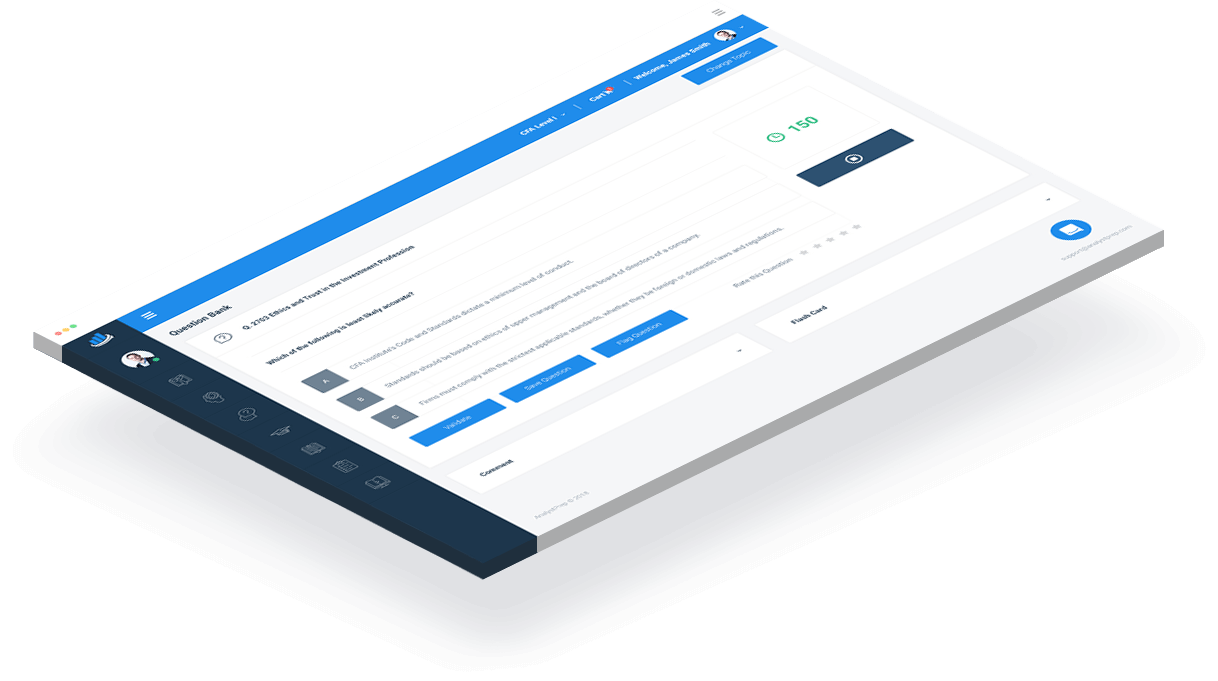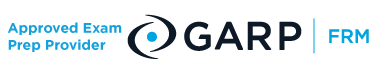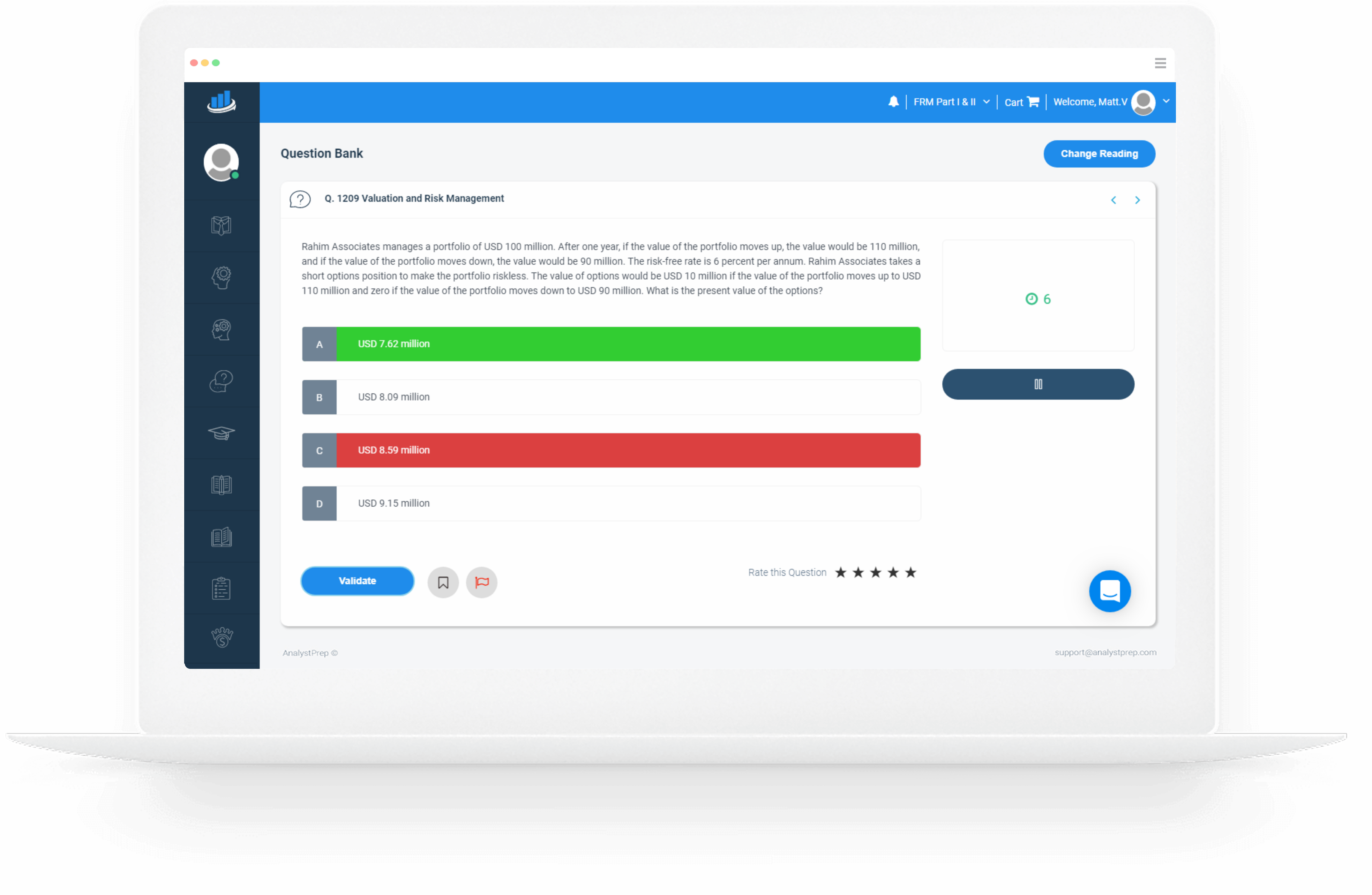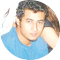Study Materials for CFA®, FRM®, Actuarial and GMAT® Exams

### Get access to 2,000 exam-style FRM part 1 questions# FRM Question Bank

AnalystPrep’s FRM part I practice questions reflect the difficulty and style of the live FRM exam part I. We provide you with a tailored, exam-centered question bank designed to teach you all the essentials of the topics that will make up the test curriculum. The question bank undergoes regular updates to incorporate the latest curriculum changes.

Some students like to attempt the questions from the relevant part of the Question Bank as soon as they have read the corresponding study notes or watched our video lessons. Others use the Question Bank at the revision stage. Our advice is to try out various approaches and pick the one that best suits you.### Quality and Quantity

The difference between success and failure in the FRM exam part I boils down to the quality of preparation materials used by candidates. The market is not short of options, but you’ve got to prepare with the best to pass. The exam is no joke, and you’re bound to hear both success and failure stories after every exam session. At AnalystPrep, we strive to give you all the tools you need to emerge successfully and put you on a path to glory. Our questions are repeatedly curated by certified FRM professionals.

A question and answer bank doesn’t mean you will revise and practice alone. Our expert instructors are always on hand to supplement the solutions provided with additional tips and tricks.

# Free FRM Part 1 Practice Questions

## Question 1

Foundations of Risk Management

In the lead-up to the 2007/2009 financial crisis, Lehman Brothers had positioned itself as the leading institution in the mortgage-backed securities market. Which of the following best explains why the firm failed so spectacularly despite boasting huge amounts of capital?

A) The firm was highly leveraged, reducing its ability to absorb losses

B) A large number of the firm’s mortgage-backed securities were built upon sub-prime mortgage assets

C) The firm was considered too big to fail

D) A lack of confidence among investors which in turn led to a lack of funding

The correct answer is: A)

All of the above statements explain one aspect or the other about Lehman Brothers which eventually contributed to the firm’s failure. However, the firm’s main reason for failing can be traced down to the relationship between leverage and illiquidity. To fund its aggressive growth strategy, Lehman Brothers resorted to extreme leverage that far surpassed its capacity to repay. The firm took on huge amounts of short-term debt to fund long-term assets, exposing itself to serious liquidity problems. Too much debt meant that the firm could not absorb losses when the housing bubble burst.

## Question 2

Financial Markets and Products

Alex Warner works as the senior derivatives manager at Warner Traders Limited. He’s currently managing a 120 million portfolio consisting of medium to large-cap U.S. equity. Warner wishes to lock up profit made during a recent rally and intends to use the S&P 500 futures with a multiplier of 250. At the moment, the S&P 500 and its futures are trading at USD 4,300 and USD 4,360, respectively, and Warner would like to hedge three-quarters of his market exposure over a three-month period. Warner’s portfolio has a correlation of 0.8 with the S&P 500 index futures, and the volatilities of the equity fund and the index futures are 0.55 and 0.50, respectively. To achieve his objective, Warner should: A) Sell 73 futures contracts B) Sell 61 futures contracts C) Buy 61 futures contracts D) Buy 73 futures contracts The correct answer is: A) The expression for the optimal hedge ratio, h, is as follows: $$h=\rho\frac{\sigma_S}{\sigma_F}$$ where: $$ρ$$ = correlation between the portfolio and the index futures $$\sigma_s$$ = volatility of the portfolio $$\sigma_F$$ = volatility of the index futures Thus, $$h=0.8\times\frac{0.55}{0.5}=0.88$$ The optimal number of futures contracts needed, N, is determined as follows: $$N=h\times\frac{Q_S}{Q_F}$$ where: where: $$h$$ = hedge ratio $$Q_S$$ = Size of the position to be hedged $$Q_F$$ = Number of units of the asset underlying one futures contract, Furthermore, note that only three-quarters of the total exposure needs to be hedged, i.e., 0.75 × USD 120 million = USD 90 million. Thus, $$N=0.88\times\frac{90,000,000}{4,360\times250}=72.67\approx73$$ Option B is incorrect. The optimal hedge ratio, h, is wrongly calculated as $$\rho\frac{\sigma_F}{\sigma_S}$$ C and D are incorrect. Since Warner has a long position in the underlying asset, he needs to take a short position (sell) to hedge the portfolio. ## Question 3 Quantitative Analysis A random sample of 50 FRM exam candidates was found to have an average I.Q. of 125. The standard deviation among candidates is known (approximately 20). Assuming that I.Q.s follow a normal distribution, carry out a statistical test (5% significance level) to determine whether the average I.Q. of FRM candidates is greater than 120. Compute the test statistic and give a conclusion. A) Test statistic: 1.768; Reject $$H_0$$ B) Test statistic: 2.828; Reject $$H_0$$ C) Test statistic: 1.768; Fail to reject $$H_0$$ D) Test statistic: 1.0606; Fail to reject $$H_0$$ The correct answer is: A) The first step: Formulate H0 and H1 \begin{align}H_0: μ &= 120\\ H1:μ & > 120\end{align} Note that this is a one-sided test because H1 explores a change in one direction only Under $$H_0$$, $$\frac{(\bar{X} – 120)}{(σ/\sqrt{n}) } \sim N(0,1)$$ Next, compute the test statistic: $$= \frac{(125 – 120)}{(20/\sqrt{50})} = 1.768$$ Next, we know that $$P(Z > 1.6449) = 0.05$$, which means our critical value is the upper 5% point of the normal distribution, i.e., 1.6449. Since 1.768 is greater than 1.6449, it lies in the rejection region. As such, we have sufficient evidence to reject H0 and conclude that the average I.Q. of FRM candidates is indeed greater than 120. Alternatively, we could go the “p-value way.” The p-value is the smallest level of significance at which we can reject the null hypothesis $$\text{p-value} = P(Z > 1.768) = 1 – P(Z < 1.768) = 1 – 0.96147 = 0.03853 \ \text{or}\ 3.853\%$$ This probability is less than 5%, meaning that there’s sufficient evidence against H0. This approach leads to the same conclusion – that the average I.Q. of FRM candidates is greater than 120. ## Question 4 Valuation and Risk Models A newly employed quantitative analyst at the risk management department of a systematically important bank (SIB) has developed a new risk measure (p) that could prove applicable to a wide range of risks at the bank. During validation and testing by an independent panel, she is asked to demonstrate that her measure is a coherent risk measure. She proceeds to formulate the following values: • There are two portfolios – Y and Z • y and z are state-contingent values of portfolios Y and Z, respectively • P(y) and p(z) are the risk measures of the two portfolios • b and c are constants where b > 0 • n is an amount in dollars Which of the following equations effectively rules out p as a coherent risk measure? A) P(y + z) ≤ p(y) + p(z) B) P(by) ≤ bP(y) C) P(y) ≥ p(z) if y ≤ z D) $$\text{P(y + c) = p(z) – c}$$ The correct answer is: B) Equation B demonstrates that the risk measure does not satisfy the homogeneity property. The homogeneity property requires that $$\text{P(by) = bP(y)}$$ Interpretation: Increasing the size of a portfolio by a factor b should result in a proportionate scale in its risk measure. For example, if the value of b is 2, then the risk of the portfolio will be doubled. Option A correctly demonstrates the subadditivity property. Interpretation: If we add two portfolios together, the total risk can’t get any worse than adding the two risks separately. Option C correctly demonstrates the monotonicity property. Interpretation: If a portfolio has systematically lower values than another, it must have a greater risk in each state of the world. In other words, if a portfolio gives undesirables results than the others, then it must be riskier. ## Question 5 Valuation and Risk Models A trader holds options on a U.K stock that doesn’t pay dividends. The stock’s current price is USD 300, and the options will expire in a year. All of the options have a strike price of USD 280. Which of the following is closest to the Black-Scholes values of these options? Assumptions: • Risk-free rate (continuously compounded) = 5% • $$Nd_1$$ = 0.7327 • $$Nd_2$$ = 0.6164 A) Value of the American call option is USD 55.34 and that of the European put is USD 21.98 B) Value of the American call option is USD 21.98 and that of the European put is USD 0.00 C) Value of the American call option is USD 55.34 and that of the European put is USD 0.00 D) Value of the American call option is USD 21.98 and that of the European put is USD 55.34 The correct answer is: A) For European options, $$\text{Price}_{call}=P_0N\left(d_1\right)-Xe^{-rt}N\left(d_2\right)$$ $$\text{Price}_{put}=Xe^{-rt}\left(1-N\left(d_2\right)\right)-P_0\left(1-N\left(d_1\right)\right)$$ where: $$P_0$$ = spot price of the stock $$X$$ = options’ strike price $$r$$ = risk-free rate $$t$$ = time to expiration of the options $$N(.)$$ = cumulative normal distribution function Thus, $$\text{Price}_{call}=300\times0.7317-280\times0.9512\times0.6164=55.34$$ $$\text{Price}_{put}=280\times0.9512\times0.3836-300\times0.2673=21.98$$ As seen from above, the value of each European call option is USD 55.34, while that of each put is USD 21.98. We also know that the value of an American option will never be less than that of an equivalent European option (same underlying, same expiration date, same strike), as it offers the same rights and more. We also know that the difference between American and European options is the early exercise feature of American-style options. But we also know that in the absence of dividends, an American call option has the same value as an equivalent European call option because there is no incentive to exercise early, and therefore the early exercise feature has no value. Thus, the only correct option is A.### FRM®Part 1 Our FRM® study packages start as low as249 with a one exam Practice package.

Our Learn + Practice package include study notes and video lessons for $399. Combine FRM Part I and FRM Part II Learn + Practice for$699. This package includes unlimited ask-a-tutor questions and lifetime access with curriculum updates each year at no extra cost.

##### FRM Part 1 Practice Package
###### / lifetime access
• Question Bank (Part 1 & Part 2 )
• CBT Mock Exams (Part 1 & Part 2 )
• Formula Sheets (Part 1 & Part 2)
• Performance Tracking Tools
• Video Lessons (Part 1 & Part 2 )
• Study Notes (Part 1 & Part 2 )

### Testimonials

“Thanks to your program I passed the first level of the CFA exam, as I got my results today. You guys are the best. I actually finished the exam with 45 minutes left in [the morning session] and 15 minutes left in [the afternoon session]… I couldn’t even finish with more than 10 minutes left in the AnalystPrep mock exams so your exams had the requisite difficulty level for the actual CFA exam.”###### James B.

“I loved the up-to-date study materials and Question bank. If you wish to increase your chances of CFA exam success on your first attempt, I strongly recommend AnalystPrep.”###### Jose Gary

“Before I came across this website, I thought I could not manage to take the CFA exam alongside my busy schedule at work. But with the up-to-date study material, there is little to worry about. The Premium package is cheaper and the questions are well answered and explained. The question bank has a wide range of examinable questions extracted from across the whole syllabus. Thank you so much for helping me pass my first CFA exam.”###### Brian Masibo

Good Day!

I cleared FRM Part I (May-2018) with 1.1.1.1. Thanks a lot to AnalystPrep and your support.

Regards,@AnalystPrep provided me with the necessary volume of questions to insure I went into test day having in-depth knowledge of every topic I would see on the exams.”###### Justin T.

“Great study materials and exam-standard questions. In addition, their customer service is excellent. I couldn’t have found a better CFA exam study partner.”###### Joshua Brown

“I bought their FRM Part 1 package and passed the exam. Their customer support answered all of my questions when I had problems with what was written in the curriculum. I’m planning to use them also for the FRM Part 2 exam and Level I of the CFA exam.”###### Zubair Jatoi

“I bought the FRM exam premium subscription about 2 weeks ago. Very good learning tool. I contacted support a few times for technical questions and Michael was very helpful.”